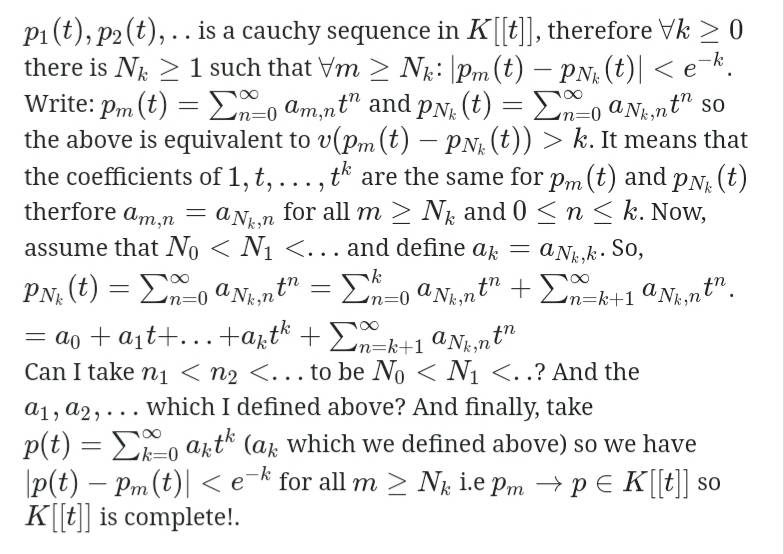# Completeness of the formal power series and valued fields

• A
aalma
TL;DR Summary
Trying to understand the completeness of K[[x]] and how to show that every cauchy sequence in it has a limit in K[[x]].
I had difficulty showing this no matter what I tried in (a) I am not getting it. Here for p(t) in K[[t]] : ## |p|=e^{-v(p)} ## where v(p) is the minimal index with a non-zero coiefficient.
I said that p_i is a cauchy sequence so, for every epsilon>0 there exists a natural N such that for all i,j>N we have
## |p_i(t)-p_j(t)|<epsilon ##, which is equivalent to that
## v(p_i(t)-p_j(t))>e^{-epsilon} ##.
But could not see how it helps here!.

Any clarifications would be great

#### Attachments

Last edited:

•